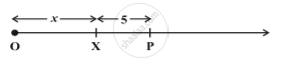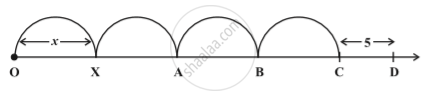Share

# Concept of Expression

#### description

• Number line and an expression

#### notes

Consider the expression x + 5. Let us say the variable x has a position X on the number line;X may be anywhere on the number line, but it is definite that the value of x + 5 is given by a point P, 5 units to the right of  X. Similarly, the value of x – 4 will be 4 units to the left of X and so on.
What about the position of 4x and 4x + 5?The position of 4x will be point C; the distance of C from the origin will be four times the distance of X from the origin. The position D of 4x + 5 will be 5 units to the right of C.

S